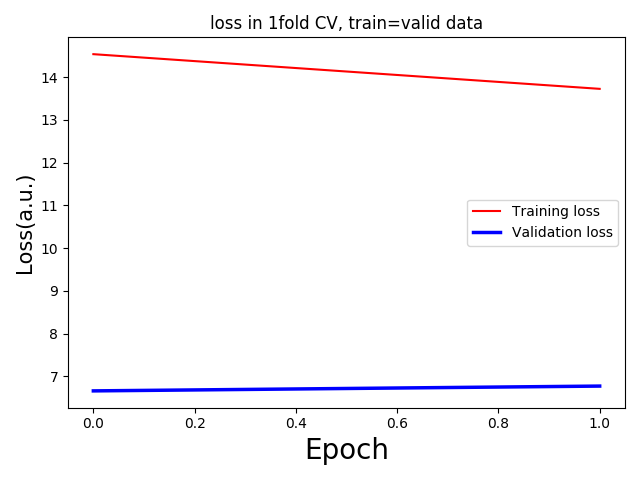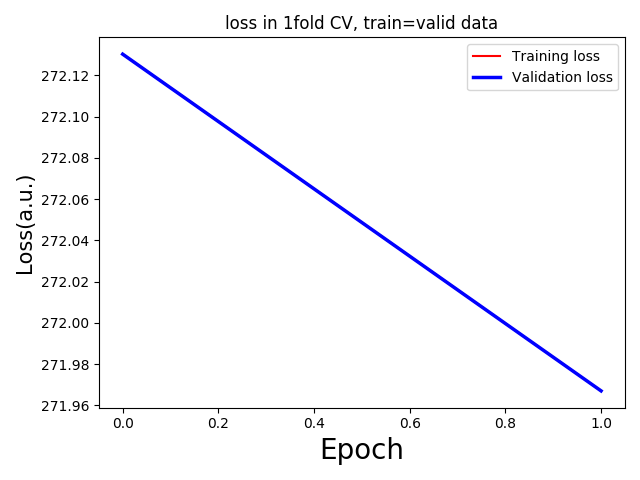# Why different validation and train loss for the same data

Dear Altruists,
I am running some regression analysis with 3D MRI data. But I am getting too low validation loss with respect to the training loss. For 5 fold validation, each having only one epoch(as a trial) I am getting the following loss curves:To debug the issue, I used the same input and target for training and validation setups in my codes.

For building model:

``````def build_model():
torch.cuda.manual_seed(1)
model = ResNet3D().to(device)
model.apply(weights_init)
Criterion = nn.MSELoss().cuda()
return model, Optimizer, Criterion
``````

I will run the `build_model` function for every fold that is my plan.

``````Net, Optimizer, Criterion = build_model()
``````

Training section:

``````Net.train()
input = sample               #just taking one sample for loss calculation
input = input.unsqueeze(0).float().to(device)   #torch.Size([1, 86, 110, 78])
target = sample
target = target.unsqueeze(0).float().to(device) #torch.Size([1, 256])

output = Net(input)   #torch.Size([1, 256])

loss = Criterion(output,target)
print("Loss in training:",loss.item())
``````

Loss in training: 14.414422035217285

** I did not update Net weights `Optimizer.step()` or neither did back propagation `loss.backward()`

Validation section:

``````Net.eval()
outputvalid = Net(input)   #same input in same Net
lossvalid = Criterion(outputvalid,target)
print("Loss in validation: ",lossvalid.item())
``````

Loss in validation: 2.8760955333709717

What could be the reason??

1 Like

If you are using a high drop probability in `Dropout` layers, this effect is usually expected.
Alternatively, the initial running stats of the batch norm layers might be better than the actual batch statistics, although this effect would be new to me. Usually you would see a lower training loss than validation.

Could you try to call `eval()` on all batch norm and dropout layers during training and check the losses again?

1 Like

Hi @ptrblck
I am not using any dropout layers. The architecture I am using can be seen below:

``````ResNet3D(
(strConv1): Conv3d(64, 64, kernel_size=(3, 3, 3), stride=(2, 2, 2), padding=(1, 1, 1))
(strConv2): Conv3d(128, 128, kernel_size=(3, 3, 3), stride=(2, 2, 2), padding=(1, 1, 1))
(strConv3): Conv3d(256, 256, kernel_size=(3, 3, 3), stride=(2, 2, 2), padding=(1, 1, 1))
(conv_block1_32): ConvBlock(
(conv1): Conv3d(1, 32, kernel_size=(3, 3, 3), stride=(1, 1, 1), padding=(1, 1, 1))
(bn1): BatchNorm3d(32, eps=1e-05, momentum=0.1, affine=True, track_running_stats=True)
(conv2): Conv3d(32, 32, kernel_size=(3, 3, 3), stride=(1, 1, 1), padding=(1, 1, 1))
(bn2): BatchNorm3d(32, eps=1e-05, momentum=0.1, affine=True, track_running_stats=True)
)
(conv_block32_64): residualUnit(
(conv1): Conv3d(32, 64, kernel_size=(3, 3, 3), stride=(1, 1, 1), padding=(1, 1, 1))
(bn1): BatchNorm3d(64, eps=1e-05, momentum=0.1, affine=True, track_running_stats=True)
(conv2): Conv3d(64, 64, kernel_size=(3, 3, 3), stride=(1, 1, 1), padding=(1, 1, 1))
(bn2): BatchNorm3d(64, eps=1e-05, momentum=0.1, affine=True, track_running_stats=True)
(convX): Conv3d(32, 64, kernel_size=(1, 1, 1), stride=(1, 1, 1))
(bnX): BatchNorm3d(64, eps=1e-05, momentum=0.1, affine=True, track_running_stats=True)
)
(conv_block64_128): residualUnit(
(conv1): Conv3d(64, 128, kernel_size=(3, 3, 3), stride=(1, 1, 1), padding=(1, 1, 1))
(bn1): BatchNorm3d(128, eps=1e-05, momentum=0.1, affine=True, track_running_stats=True)
(conv2): Conv3d(128, 128, kernel_size=(3, 3, 3), stride=(1, 1, 1), padding=(1, 1, 1))
(bn2): BatchNorm3d(128, eps=1e-05, momentum=0.1, affine=True, track_running_stats=True)
(convX): Conv3d(64, 128, kernel_size=(1, 1, 1), stride=(1, 1, 1))
(bnX): BatchNorm3d(128, eps=1e-05, momentum=0.1, affine=True, track_running_stats=True)
)
(conv_block128_256): residualUnit(
(conv1): Conv3d(128, 256, kernel_size=(3, 3, 3), stride=(1, 1, 1), padding=(1, 1, 1))
(bn1): BatchNorm3d(256, eps=1e-05, momentum=0.1, affine=True, track_running_stats=True)
(conv2): Conv3d(256, 256, kernel_size=(3, 3, 3), stride=(1, 1, 1), padding=(1, 1, 1))
(bn2): BatchNorm3d(256, eps=1e-05, momentum=0.1, affine=True, track_running_stats=True)
(convX): Conv3d(128, 256, kernel_size=(1, 1, 1), stride=(1, 1, 1))
(bnX): BatchNorm3d(256, eps=1e-05, momentum=0.1, affine=True, track_running_stats=True)
)
(conv1d): ConvBlock1D(
(conv1): Conv1d(1, 2, kernel_size=(3,), stride=(1,), padding=(1,))
(bn1): BatchNorm1d(2, eps=1e-05, momentum=0.1, affine=True, track_running_stats=True)
)
(fc1): Linear(in_features=788480, out_features=256, bias=True)
(fc2): Linear(in_features=256, out_features=256, bias=True)
)
``````

I am using the following weight initialization:

``````def weights_init(m):
if isinstance(m, nn.Conv3d or nn.Conv1d): #nn.Conv3d
torch.nn.init.xavier_uniform_(m.weight.data, init.calculate_gain('relu'))
m.bias.data.fill_(0)
# torch.nn.init.xavier_uniform_(m.bias.data)
elif isinstance(m, nn.BatchNorm3d):
m.weight.data.normal_(mean=1.0, std=0.02)
m.bias.data.fill_(0)
elif isinstance(m, nn.Linear):
m.weight.data.normal_(0.0, 0.02)
m.bias.data.fill_(0)
``````

Just to clarify how do I call eval on only batchnorm layers?

`Net.conv_block32_64.bn1.eval()`

Something like above?

1 Like

Yes, that should work.
A more convenient method would be:

``````def set_bn_eval(m):
if isinstance(m, nn.modules.batchnorm._BatchNorm):
m.eval()

model.apply(set_bn_eval)
``````

EDIT: Note that in your weight init code you are not initializing `nn.BatchNorm1d` using the specified method, but only `nn.BatchNorm3d`. My condition should work on both layer types, in case you need it.

2 Likes

I ran the codes as below making sure that the batchnorm layers in eval mode(as the code you shared)

``````    train_dataset = Subset(dataset, train_idx)
valid_dataset = Subset(dataset, valid_idx)
for epoch in range(max_epoch):
print("training...")
epoch_wise_loss = 0
epoch_accuracy = 0
running_time_batch = 0
time_batch_start = time.time()
Net.train()
Net.apply(set_bn_eval)
``````

But I think the train loss increased and validation loss did not change or even reduced.Then I changed the `valid_loader` also with `train_dataset` to keep the train and validation is the same data. And removed the `Optimizer.zero_grad()` and `Optimizer.step()` from the train section. This time the train and validation losses are almost the same which kind of makes sense.

Why is validation loss decreasing when I use the Optimizer functions?

``````    train_loader = data.DataLoader(train_dataset, batch_size=batchsize, shuffle=True)
for epoch in range(max_epoch):
print("training...")
epoch_wise_loss = 0
epoch_accuracy = 0
running_time_batch = 0
time_batch_start = time.time()
Net.train()
Net.apply(set_bn_eval)
time_compute_start = time.time()
mr = sample.float().to(device)  #torch.Size([2, 1, 86, 110, 78])

shimVal = sample.float().to(device)  #torch.Size([2, 512])
out = Net(mr)  #torch.Size([2, 512])
Loss = Criterion(shimVal, out)
print("Batch train loss:", Loss.item())
batch_accuracy = accuracy(shimVal, out)
Loss.backward()
# Optimizer.step()
epoch_wise_loss += Loss.item()
mean_loss = epoch_wise_loss/(tBatch_idx+1)  #avg loss till current batch
``````

Did you randomly split the data import the training and validation sets or are you using data e.g. from other data domains?

If your validation loss decreasing even though you don’t call `optimizer.step()`?

I am experimenting with cross-validation.
For splitting the train and validation set I am using the `Subset` class of PyTorch.

``````class Subset(Dataset):
"""
Subset of a dataset at specified indices.
Arguments:
dataset (Dataset): The whole Dataset
indices (sequence): Indices in the whole set selected for subset
"""
def __init__(self, dataset, indices):
self.dataset = dataset
self.indices = indices

def __len__(self):
if self.indices.shape == ():
print('this happens: Subset')
return 1
else:
return len(self.indices)

def __getitem__(self, idx):
return self.dataset[self.indices[idx]]
``````

`num_val_samples = len(dataset)//k`

``````for i in range(k):
print('Processing fold: ', i + 1)
"""%%%% Initiate new model %%%%""" #in every fold
# torch.cuda.manual_seed(1)
# model = ResNet3D().to(device)
# model.apply(weights_init)
# Criterion = nn.MSELoss()
# Criterion = Criterion.cuda()
Net, Optimizer, Criterion = build_model()
"""%%%% Split train and validation sets %%%%"""
valid_idx = np.arange(len(dataset))[i * num_val_samples:(i + 1) * num_val_samples]
print('Valid fold indices:', valid_idx + 1)
train_idx = np.concatenate([np.arange(len(dataset))[:i * num_val_samples], np.arange(len(dataset))[(i + 1) * num_val_samples:]], axis=0)
print('Train fold indices:', train_idx+1)
train_dataset = Subset(dataset, train_idx)
valid_dataset = Subset(dataset, valid_idx)
``````

Without using the `Optimizer.step()` I am getting the following loss in 1 fold cv:I did not do anything to the validation section. So it was as before:

``````        print('validating...')
Net.eval()
epoch_wise_loss = 0
# for vBatch_idx, sample in enumerate(valid_loader):
# Net.eval()
mr = sample.float().to(device)
# print("Valid input mean:", mr.mean())
shimVal = sample.float().to(device)
# print("Valid target mean:", shimVal.mean())
out = Net(mr)
Loss = Criterion(shimVal, out)
print("Batch val loss:", Loss.item())
batch_accuracy = accuracy(shimVal, out)
epoch_wise_loss += Loss.item()
mean_loss = epoch_wise_loss / (vBatch_idx + 1)
``````

These are all without setting batchnorm to eval mode. The next is setting batchnorm to eval mode as following:

``````Net.train()
Net.apply(set_bn_eval)
``````Train and validation loss are exactly the same and increase as you can see from the graph above.

Hi! Did you figure out what was wrong? I’m having the same issue and it is driving me crazy.